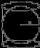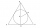# Ratio + sphere - math problems

#### Number of problems found: 10

• Cube, cuboid, and sphereVolumes of a cube and a cuboid are in ratio 3: 2. Volumes of sphere and cuboid are in ratio 1: 3. At what rate are the volumes of cube, cuboid, and sphere?
• Sphere cutsAt what distance from the center intersects sphere with radius R = 56 plane, if the cut area and area of the main sphere circle is in ratio 1/2.The radius of the sphere we reduce by 1/3 of the original radius. How much percent does the volume and surface of the sphere change?
• Volume of sphereHow many times does the volume of a sphere increase if its radius increases 2 ×?
• Inscribed sphereHow many % of the volume of the cube whose edge is 6 meters long is a volume of a sphere inscribed in that cube?
• Equilateral cylinderA sphere is inserted into the rotating equilateral cylinder (touching the bases and the shell). Prove that the cylinder has both a volume and a surface half larger than an inscribed sphere.
• Sphere in coneA sphere is inscribed in the cone (the intersection of their boundaries consists of a circle and one point). The ratio of the surface of the ball and the contents of the base is 4: 3. A plane passing through the axis of a cone cuts the cone in an isosceleHow many times does the surface of a sphere decrease if we reduce its radius twice?
• Volume ratioCalculate the volume ratio of balls circumscribed (diameter r) and inscribed (diameter ϱ) into an equilateral rotating cone.
• BallsPing pong balls have a diameter of approximately 5.1 cm. It sold in boxes of 10 pieces: each box has a cuboid shape with a square base. The balls touch the walls of the box. Calculate what portion of the internal volume of the box is filled with balls.

We apologize, but in this category are not a lot of examples.
Do you have an interesting mathematical word problem that you can't solve it? Submit a math problem, and we can try to solve it.

We will send a solution to your e-mail address. Solved examples are also published here. Please enter the e-mail correctly and check whether you don't have a full mailbox.

Please do not submit problems from current active competitions such as Mathematical Olympiad, correspondence seminars etc...

Check out our ratio calculator. Ratio - math word problems. Sphere Problems.